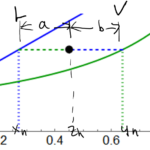LearnChemE

#### Lever Rule: Screencast

Use a mass balance to derive the lever rule, which determines the amounts of liquid and vapor in equilibrium, given the overall mole fraction and the mole fractions in each phase for a binary mixture.

We suggest you list the important points in this screencast as a way to increase retention.

##### Important Equations:The lever rule for a binary system with vapor and liquid in equilibrium:

$aL = bV$

where $$a$$ and $$b$$ are the distances in the figure above and $$L$$ and $$V$$ are the number of moles of liquid and vapor, respectively.

The lever rule can also be written as:

$(z_h – x_h)L = (y_h -z_h)V$

where$$x_h$$ and $$y_h$$ are the liquid and vapor fractions, respectively, and $$z_h$$ is the overall mole fraction of the mixture.